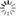10% off your first order! Code: FIRST10

Claim a Pumpkin Spice FREE on orders above Rs. 999

Free Shipping on orders over Rs. 500

10% off your first order. Use code FIRST10

Claim a Pumpkin Spice FREE on orders above Rs. 999

Free Shipping on orders over Rs. 500

Currencies
My CartProducts

Rs. 225.00 Rs. 450.00

Buy 2 for Rs. 450 Rs. 425

Rs. 250.00 Rs. 500.00

Buy 2 for Rs. 500 Rs. 475

Rs. 250.00 Rs. 500.00

Buy 2 for Rs. 500 Rs. 475

Rs. 300.00 Rs. 350.00

MRP Rs. 350 Rs. 300

Rs. 300.00 Rs. 350.00

MRP Rs. 350 Rs. 300

Rs. 500.00 Rs. 700.00

MRP Rs. 975 Rs. 500

Rs. 800.00 Rs. 1,100.00

MRP Rs. 1100 Rs. 800

Rs. 300.00 Rs. 600.00

Buy 2 for Rs. 600 Rs. 550

Rs. 250.00 Rs. 500.00

Buy 2 for Rs. 500 Rs. 475

Rs. 400.00 Rs. 800.00

Buy 2 for Rs. 800 Rs. 650

Rs. 250.00 Rs. 500.00

Buy 2 for Rs. 500 Rs. 475

Rs. 300.00

Available only in Mumbai

Rs. 399.00 Rs. 798.00

Buy 2 for Rs. 798 Rs. 748

Rs. 450.00 Rs. 475.00

MRP Rs. 475

Rs. 550.00 Rs. 725.00

MRP Rs. 725

Rs. 700.00 Rs. 975.00

MRP Rs. 975

Rs. 225.00 Rs. 450.00

Buy 2 for Rs. 450 Rs. 425

Rs. 250.00 Rs. 500.00

Buy 2 for Rs. 500 Rs. 475

Rs. 250.00 Rs. 500.00

Buy 2 for Rs. 500 Rs. 475

Rs. 250.00 Rs. 500.00

Buy 2 for Rs. 500 Rs. 475

Rs. 399.00 Rs. 798.00

Buy 2 for Rs. 798 Rs. 748

Rs. 300.00 Rs. 600.00

Buy 2 for Rs. 600 Rs. 550

Rs. 250.00 Rs. 500.00

Buy 2 for Rs. 500 Rs. 475

Rs. 300.00 Rs. 350.00

MRP Rs. 350 Rs. 300

Rs. 300.00 Rs. 350.00

MRP Rs. 350 Rs. 300

Rs. 400.00 Rs. 800.00

Buy 2 for Rs. 800 Rs. 650

Rs. 500.00 Rs. 700.00

MRP Rs. 975 Rs. 500

Rs. 800.00 Rs. 1,100.00

MRP Rs. 1100 Rs. 800

Rs. 500.00 Rs. 700.00

MRP Rs. 975 Rs. 500

Rs. 800.00 Rs. 1,100.00

MRP Rs. 1100 Rs. 800

Rs. 450.00 Rs. 475.00

MRP Rs. 475

Rs. 550.00 Rs. 725.00

MRP Rs. 725

Rs. 700.00 Rs. 975.00

MRP Rs. 975

Rs. 1,000.00 Rs. 1,125.00

MRP Rs. 1125

Rs. 1,750.00 Rs. 2,025.00

MRP Rs. 2025

Rs. 600.00 Rs. 650.00

MRP Rs. 650

Rs. 475.00 Rs. 500.00

MRP Rs. 500

Rs. 950.00 Rs. 1,050.00

MRP Rs. 1050

Rs. 750.00 Rs. 825.00

MRP Rs. 825

Rs. 300.00

Available only in Mumbai

Rs. 750.00